# Insertion in a B+ tree

Prerequisite: Introduction of B+ trees

In this article, we will discuss that how to insert a node in B+ Tree. During insertion following properties of B+ Tree must be followed:

• Each node except root can have a maximum of M children and at least ceil(M/2) children.
• Each node can contain a maximum of M – 1 keys and a minimum of ceil(M/2) – 1 keys.
• The root has at least two children and atleast one search key.
• While insertion overflow of the node occurs when it contains more than M – 1 search key values.

Here M is the order of B+ tree.

### Steps for insertion in B+ Tree

1. Every element is inserted into a leaf node. So, go to the appropriate leaf node.
2. Insert the key into the leaf node in increasing order only if there is no overflow. If there is an overflow go ahead with the following steps mentioned below to deal with overflow while maintaining the B+ Tree properties.

### Properties for insertion B+ Tree

Case 1: Overflow in leaf node

1. Split the leaf node into two nodes.
2. First node contains ceil((m-1)/2) values.
3. Second node contains the remaining values.
4. Copy the smallest search key value from second node to the parent node.(Right biased)

Below is the illustration of inserting 8 into B+ Tree of order of 5: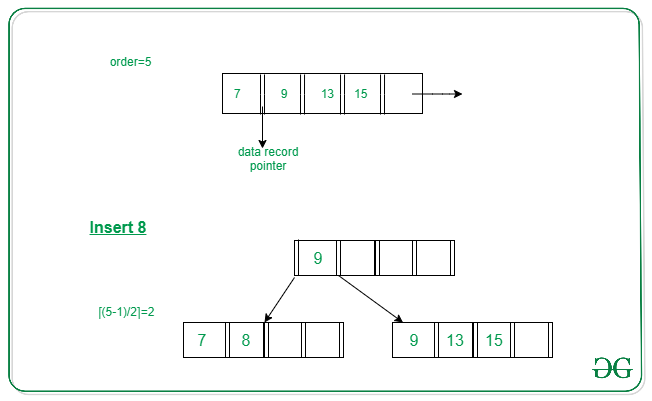Case 2: Overflow in non-leaf node

1. Split the non leaf node into two nodes.
2. First node contains ceil(m/2)-1 values.
3. Move the smallest among remaining to the parent.
4. Second node contains the remaining keys.

Below is the illustration of inserting 15 into B+ Tree of order of 5: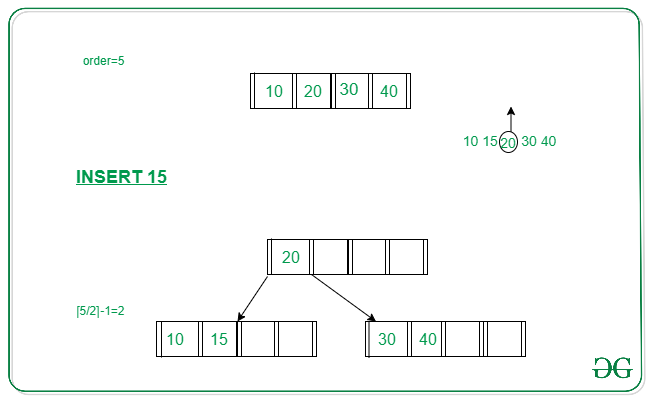## Example to illustrate insertion on a B+ tree

Problem: Insert the following key values 6, 16, 26, 36, 46 on a B+ tree with order = 3.
Solution:
Step 1: The order is 3 so at maximum in a node so there can be only 2 search key values. As insertion happens on a leaf node only in a B+ tree so insert search key value 6 and 16 in increasing order in the node. Below is the illustration of the same: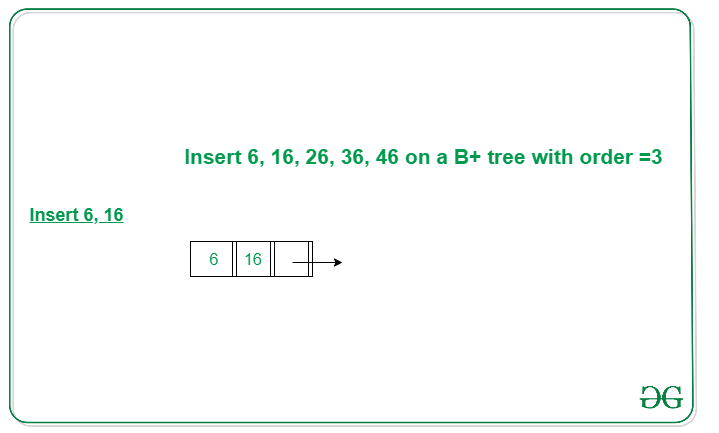Step 2: We cannot insert 26 in the same node as it causes an overflow in the leaf node, We have to split the leaf node according to the rules. First part contains ceil((3-1)/2) values i.e., only 6. The second node contains the remaining values i.e., 16 and 26. Then also copy the smallest search key value from the second node to the parent node i.e., 16 to the parent node. Below is the illustration of the same: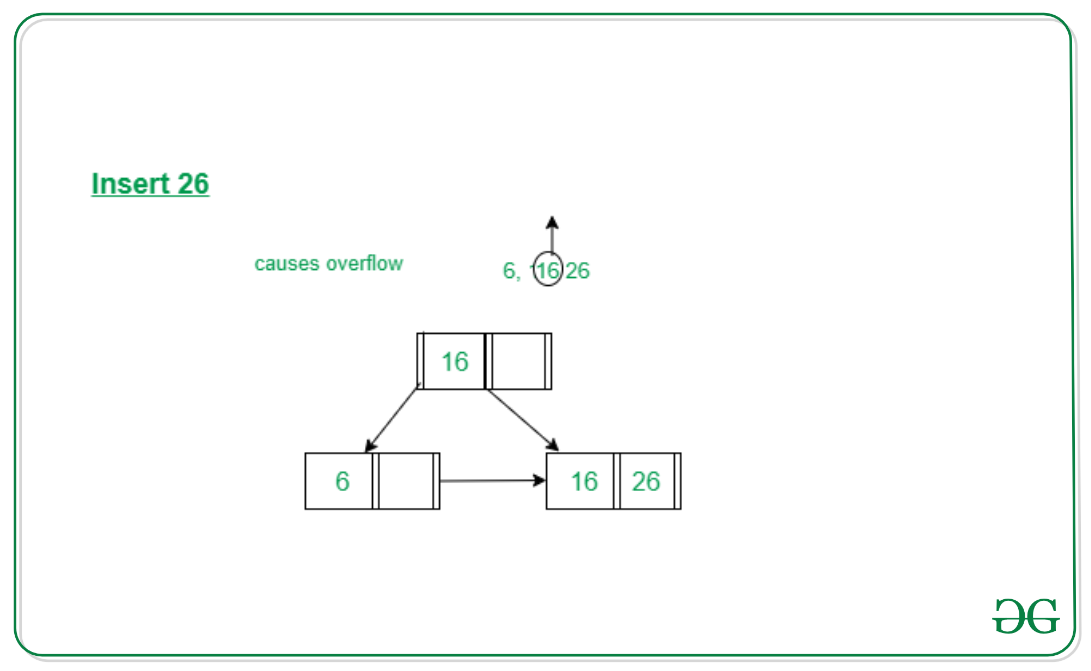Step 3: Now the next value is 36 that is to be inserted after 26 but in that node, it causes an overflow again in that leaf node. Again follow the above steps to split the node. First part contains ceil((3-1)/2) values i.e., only 16. The second node contains the remaining values i.e., 26 and 36. Then also copy the smallest search key value from the second node to the parent node i.e., 26 to the parent node. Below is the illustration of the same:
The illustration is shown in the diagram below.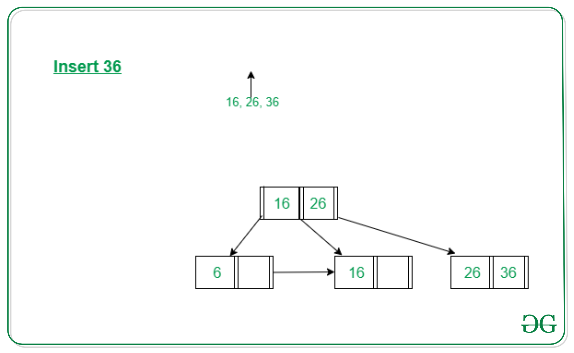Step 4: Now we have to insert 46 which is to be inserted after 36 but it causes an overflow in the leaf node. So we split the node according to the rules. The first part contains 26 and the second part contains 36 and 46 but now we also have to copy 36 to the parent node but it causes overflow as only two search key values can be accommodated in a node. Now follow the steps to deal with overflow in the non-leaf node.
First node contains ceil(3/2)-1 values i.e. ’16’.
Move the smallest among remaining to the parent i.e ’26’ will be the new parent node.
The second node contains the remaining keys i.e ’36’ and the rest of the leaf nodes remain the same. Below is the illustration of the same: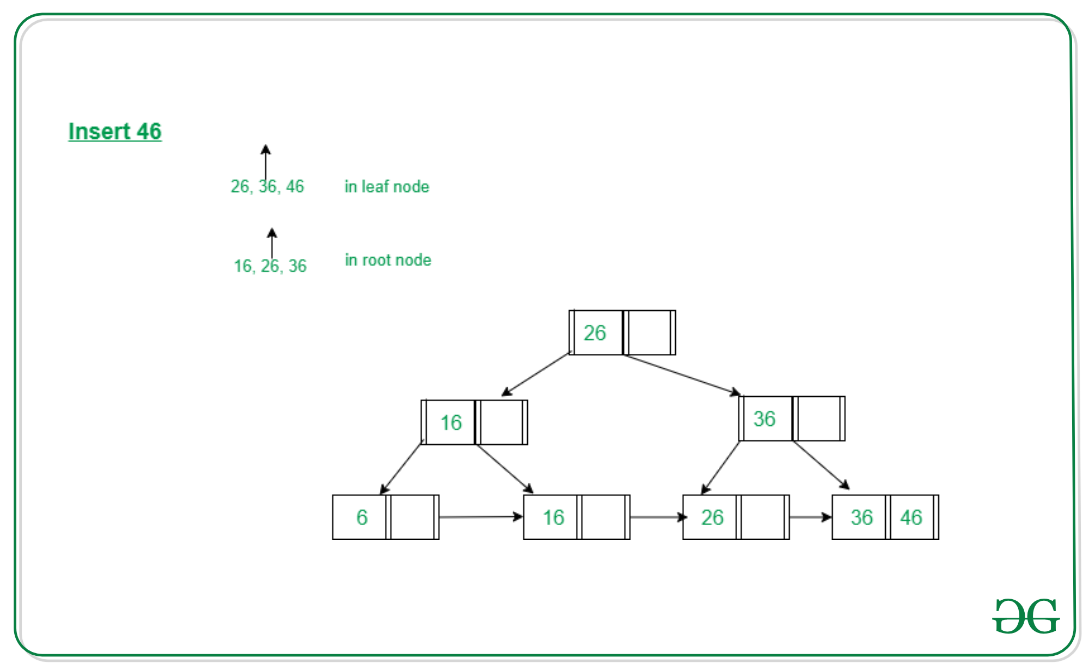Below is the implementation of insertion in the B+ tree:

## C++

 `// C++ program for implementing B+ Tree ` `#include ` `#include ` `#include ` `#include ` `using` `namespace` `std; ` `int` `MAX = 3; ` ` `  `// BP node ` `class` `Node { ` `    ``bool` `IS_LEAF; ` `    ``int` `*key, size; ` `    ``Node** ptr; ` `    ``friend` `class` `BPTree; ` ` `  `public``: ` `    ``Node(); ` `}; ` ` `  `// BP tree ` `class` `BPTree { ` `    ``Node* root; ` `    ``void` `insertInternal(``int``, ` `                        ``Node*, ` `                        ``Node*); ` `    ``Node* findParent(Node*, Node*); ` ` `  `public``: ` `    ``BPTree(); ` `    ``void` `search(``int``); ` `    ``void` `insert(``int``); ` `    ``void` `display(Node*); ` `    ``Node* getRoot(); ` `}; ` ` `  `// Constructor of Node ` `Node::Node() ` `{ ` `    ``key = ``new` `int``[MAX]; ` `    ``ptr = ``new` `Node*[MAX + 1]; ` `} ` ` `  `// Initialise the BPTree Node ` `BPTree::BPTree() ` `{ ` `    ``root = NULL; ` `} ` ` `  `// Function to find any element ` `// in B+ Tree ` `void` `BPTree::search(``int` `x) ` `{ ` ` `  `    ``// If tree is empty ` `    ``if` `(root == NULL) { ` `        ``cout << ``"Tree is empty\n"``; ` `    ``} ` ` `  `    ``// Traverse to find the value ` `    ``else` `{ ` ` `  `        ``Node* cursor = root; ` ` `  `        ``// Till we reach leaf node ` `        ``while` `(cursor->IS_LEAF == ``false``) { ` ` `  `            ``for` `(``int` `i = 0; ` `                 ``i < cursor->size; i++) { ` ` `  `                ``// If the element to be ` `                ``// found is not present ` `                ``if` `(x < cursor->key[i]) { ` `                    ``cursor = cursor->ptr[i]; ` `                    ``break``; ` `                ``} ` ` `  `                ``// If reaches end of the ` `                ``// cursor node ` `                ``if` `(i == cursor->size - 1) { ` `                    ``cursor = cursor->ptr[i + 1]; ` `                    ``break``; ` `                ``} ` `            ``} ` `        ``} ` ` `  `        ``// Traverse the cursor and find ` `        ``// the node with value x ` `        ``for` `(``int` `i = 0; ` `             ``i < cursor->size; i++) { ` ` `  `            ``// If found then return ` `            ``if` `(cursor->key[i] == x) { ` `                ``cout << ``"Found\n"``; ` `                ``return``; ` `            ``} ` `        ``} ` ` `  `        ``// Else element is not present ` `        ``cout << ``"Not found\n"``; ` `    ``} ` `} ` ` `  `// Function to implement the Insert ` `// Operation in B+ Tree ` `void` `BPTree::insert(``int` `x) ` `{ ` ` `  `    ``// If root is null then return ` `    ``// newly created node ` `    ``if` `(root == NULL) { ` `        ``root = ``new` `Node; ` `        ``root->key = x; ` `        ``root->IS_LEAF = ``true``; ` `        ``root->size = 1; ` `    ``} ` ` `  `    ``// Traverse the B+ Tree ` `    ``else` `{ ` `        ``Node* cursor = root; ` `        ``Node* parent; ` ` `  `        ``// Till cursor reaches the ` `        ``// leaf node ` `        ``while` `(cursor->IS_LEAF ` `               ``== ``false``) { ` ` `  `            ``parent = cursor; ` ` `  `            ``for` `(``int` `i = 0; ` `                 ``i < cursor->size; ` `                 ``i++) { ` ` `  `                ``// If found the position ` `                ``// where we have to insert ` `                ``// node ` `                ``if` `(x < cursor->key[i]) { ` `                    ``cursor ` `                        ``= cursor->ptr[i]; ` `                    ``break``; ` `                ``} ` ` `  `                ``// If reaches the end ` `                ``if` `(i == cursor->size - 1) { ` `                    ``cursor ` `                        ``= cursor->ptr[i + 1]; ` `                    ``break``; ` `                ``} ` `            ``} ` `        ``} ` ` `  `        ``if` `(cursor->size < MAX) { ` `            ``int` `i = 0; ` `            ``while` `(x > cursor->key[i] ` `                   ``&& i < cursor->size) { ` `                ``i++; ` `            ``} ` ` `  `            ``for` `(``int` `j = cursor->size; ` `                 ``j > i; j--) { ` `                ``cursor->key[j] ` `                    ``= cursor->key[j - 1]; ` `            ``} ` ` `  `            ``cursor->key[i] = x; ` `            ``cursor->size++; ` ` `  `            ``cursor->ptr[cursor->size] ` `                ``= cursor->ptr[cursor->size - 1]; ` `            ``cursor->ptr[cursor->size - 1] = NULL; ` `        ``} ` ` `  `        ``else` `{ ` ` `  `            ``// Create a newLeaf node ` `            ``Node* newLeaf = ``new` `Node; ` ` `  `            ``int` `virtualNode[MAX + 1]; ` ` `  `            ``// Update cursor to virtual ` `            ``// node created ` `            ``for` `(``int` `i = 0; i < MAX; i++) { ` `                ``virtualNode[i] ` `                    ``= cursor->key[i]; ` `            ``} ` `            ``int` `i = 0, j; ` ` `  `            ``// Traverse to find where the new ` `            ``// node is to be inserted ` `            ``while` `(x > virtualNode[i] ` `                   ``&& i < MAX) { ` `                ``i++; ` `            ``} ` ` `  `            ``// Update the current virtual ` `            ``// Node to its previous ` `            ``for` `(``int` `j = MAX + 1; ` `                 ``j > i; j--) { ` `                ``virtualNode[j] ` `                    ``= virtualNode[j - 1]; ` `            ``} ` ` `  `            ``virtualNode[i] = x; ` `            ``newLeaf->IS_LEAF = ``true``; ` ` `  `            ``cursor->size = (MAX + 1) / 2; ` `            ``newLeaf->size ` `                ``= MAX + 1 - (MAX + 1) / 2; ` ` `  `            ``cursor->ptr[cursor->size] ` `                ``= newLeaf; ` ` `  `            ``newLeaf->ptr[newLeaf->size] ` `                ``= cursor->ptr[MAX]; ` ` `  `            ``cursor->ptr[MAX] = NULL; ` ` `  `            ``// Update the current virtual ` `            ``// Node's key to its previous ` `            ``for` `(i = 0; ` `                 ``i < cursor->size; i++) { ` `                ``cursor->key[i] ` `                    ``= virtualNode[i]; ` `            ``} ` ` `  `            ``// Update the newLeaf key to ` `            ``// virtual Node ` `            ``for` `(i = 0, j = cursor->size; ` `                 ``i < newLeaf->size; ` `                 ``i++, j++) { ` `                ``newLeaf->key[i] ` `                    ``= virtualNode[j]; ` `            ``} ` ` `  `            ``// If cursor is the root node ` `            ``if` `(cursor == root) { ` ` `  `                ``// Create a new Node ` `                ``Node* newRoot = ``new` `Node; ` ` `  `                ``// Update rest field of ` `                ``// B+ Tree Node ` `                ``newRoot->key = newLeaf->key; ` `                ``newRoot->ptr = cursor; ` `                ``newRoot->ptr = newLeaf; ` `                ``newRoot->IS_LEAF = ``false``; ` `                ``newRoot->size = 1; ` `                ``root = newRoot; ` `            ``} ` `            ``else` `{ ` ` `  `                ``// Recursive Call for ` `                ``// insert in internal ` `                ``insertInternal(newLeaf->key, ` `                               ``parent, ` `                               ``newLeaf); ` `            ``} ` `        ``} ` `    ``} ` `} ` ` `  `// Function to implement the Insert ` `// Internal Operation in B+ Tree ` `void` `BPTree::insertInternal(``int` `x, ` `                            ``Node* cursor, ` `                            ``Node* child) ` `{ ` ` `  `    ``// If we doesn't have overflow ` `    ``if` `(cursor->size < MAX) { ` `        ``int` `i = 0; ` ` `  `        ``// Traverse the child node ` `        ``// for current cursor node ` `        ``while` `(x > cursor->key[i] ` `               ``&& i < cursor->size) { ` `            ``i++; ` `        ``} ` ` `  `        ``// Traverse the cursor node ` `        ``// and update the current key ` `        ``// to its previous node key ` `        ``for` `(``int` `j = cursor->size; ` `             ``j > i; j--) { ` ` `  `            ``cursor->key[j] ` `                ``= cursor->key[j - 1]; ` `        ``} ` ` `  `        ``// Traverse the cursor node ` `        ``// and update the current ptr ` `        ``// to its previous node ptr ` `        ``for` `(``int` `j = cursor->size + 1; ` `             ``j > i + 1; j--) { ` `            ``cursor->ptr[j] ` `                ``= cursor->ptr[j - 1]; ` `        ``} ` ` `  `        ``cursor->key[i] = x; ` `        ``cursor->size++; ` `        ``cursor->ptr[i + 1] = child; ` `    ``} ` ` `  `    ``// For overflow, break the node ` `    ``else` `{ ` ` `  `        ``// For new Interval ` `        ``Node* newInternal = ``new` `Node; ` `        ``int` `virtualKey[MAX + 1]; ` `        ``Node* virtualPtr[MAX + 2]; ` ` `  `        ``// Insert the current list key ` `        ``// of cursor node to virtualKey ` `        ``for` `(``int` `i = 0; i < MAX; i++) { ` `            ``virtualKey[i] = cursor->key[i]; ` `        ``} ` ` `  `        ``// Insert the current list ptr ` `        ``// of cursor node to virtualPtr ` `        ``for` `(``int` `i = 0; i < MAX + 1; i++) { ` `            ``virtualPtr[i] = cursor->ptr[i]; ` `        ``} ` ` `  `        ``int` `i = 0, j; ` ` `  `        ``// Traverse to find where the new ` `        ``// node is to be inserted ` `        ``while` `(x > virtualKey[i] ` `               ``&& i < MAX) { ` `            ``i++; ` `        ``} ` ` `  `        ``// Traverse the virtualKey node ` `        ``// and update the current key ` `        ``// to its previous node key ` `        ``for` `(``int` `j = MAX + 1; ` `             ``j > i; j--) { ` ` `  `            ``virtualKey[j] ` `                ``= virtualKey[j - 1]; ` `        ``} ` ` `  `        ``virtualKey[i] = x; ` ` `  `        ``// Traverse the virtualKey node ` `        ``// and update the current ptr ` `        ``// to its previous node ptr ` `        ``for` `(``int` `j = MAX + 2; ` `             ``j > i + 1; j--) { ` `            ``virtualPtr[j] ` `                ``= virtualPtr[j - 1]; ` `        ``} ` ` `  `        ``virtualPtr[i + 1] = child; ` `        ``newInternal->IS_LEAF = ``false``; ` ` `  `        ``cursor->size ` `            ``= (MAX + 1) / 2; ` ` `  `        ``newInternal->size ` `            ``= MAX - (MAX + 1) / 2; ` ` `  `        ``// Insert new node as an ` `        ``// internal node ` `        ``for` `(i = 0, j = cursor->size + 1; ` `             ``i < newInternal->size; ` `             ``i++, j++) { ` ` `  `            ``newInternal->key[i] ` `                ``= virtualKey[j]; ` `        ``} ` ` `  `        ``for` `(i = 0, j = cursor->size + 1; ` `             ``i < newInternal->size + 1; ` `             ``i++, j++) { ` ` `  `            ``newInternal->ptr[i] ` `                ``= virtualPtr[j]; ` `        ``} ` ` `  `        ``// If cursor is the root node ` `        ``if` `(cursor == root) { ` ` `  `            ``// Create a new root node ` `            ``Node* newRoot = ``new` `Node; ` ` `  `            ``// Update key value ` `            ``newRoot->key ` `                ``= cursor->key[cursor->size]; ` ` `  `            ``// Update rest field of ` `            ``// B+ Tree Node ` `            ``newRoot->ptr = cursor; ` `            ``newRoot->ptr = newInternal; ` `            ``newRoot->IS_LEAF = ``false``; ` `            ``newRoot->size = 1; ` `            ``root = newRoot; ` `        ``} ` ` `  `        ``else` `{ ` ` `  `            ``// Recursive Call to insert ` `            ``// the data ` `            ``insertInternal(cursor->key[cursor->size], ` `                           ``findParent(root, ` `                                      ``cursor), ` `                           ``newInternal); ` `        ``} ` `    ``} ` `} ` ` `  `// Function to find the parent node ` `Node* BPTree::findParent(Node* cursor, ` `                         ``Node* child) ` `{ ` `    ``Node* parent; ` ` `  `    ``// If cursor reaches the end of Tree ` `    ``if` `(cursor->IS_LEAF ` `        ``|| (cursor->ptr)->IS_LEAF) { ` `        ``return` `NULL; ` `    ``} ` ` `  `    ``// Traverse the current node with ` `    ``// all its child ` `    ``for` `(``int` `i = 0; ` `         ``i < cursor->size + 1; i++) { ` ` `  `        ``// Update the parent for the ` `        ``// child Node ` `        ``if` `(cursor->ptr[i] == child) { ` `            ``parent = cursor; ` `            ``return` `parent; ` `        ``} ` ` `  `        ``// Else recursively traverse to ` `        ``// find child node ` `        ``else` `{ ` `            ``parent ` `                ``= findParent(cursor->ptr[i], ` `                             ``child); ` ` `  `            ``// If parent is found, then ` `            ``// return that parent node ` `            ``if` `(parent != NULL) ` `                ``return` `parent; ` `        ``} ` `    ``} ` ` `  `    ``// Return parent node ` `    ``return` `parent; ` `} ` ` `  `// Function to get the root Node ` `Node* BPTree::getRoot() ` `{ ` `    ``return` `root; ` `} ` ` `  `// Driver Code ` `int` `main() ` `{ ` `    ``BPTree node; ` ` `  `    ``// Create B+ Tree ` `    ``node.insert(6); ` `    ``node.insert(16); ` `    ``node.insert(26); ` `    ``node.insert(36); ` `    ``node.insert(46); ` ` `  `    ``// Function Call to search node ` `    ``// with value 16 ` `    ``node.search(16); ` ` `  `    ``return` `0; ` `} `

Output:

```Found
```

Attention reader! Don’t stop learning now. Get hold of all the important DSA concepts with the DSA Self Paced Course at a student-friendly price and become industry ready.

My Personal Notes arrow_drop_upCheck out this Author's contributed articles.

If you like GeeksforGeeks and would like to contribute, you can also write an article using contribute.geeksforgeeks.org or mail your article to contribute@geeksforgeeks.org. See your article appearing on the GeeksforGeeks main page and help other Geeks.

Please Improve this article if you find anything incorrect by clicking on the "Improve Article" button below.